# Engineering Mechanics - Force System Resultants

## Why Engineering Mechanics Force System Resultants?

In this section you can learn and practice Engineering Mechanics Questions based on "Force System Resultants" and improve your skills in order to face the interview, competitive examination and various entrance test (CAT, GATE, GRE, MAT, Bank Exam, Railway Exam etc.) with full confidence.

## Where can I get Engineering Mechanics Force System Resultants questions and answers with explanation?

IndiaBIX provides you lots of fully solved Engineering Mechanics (Force System Resultants) questions and answers with Explanation. Solved examples with detailed answer description, explanation are given and it would be easy to understand. All students, freshers can download Engineering Mechanics Force System Resultants quiz questions with answers as PDF files and eBooks.

## Where can I get Engineering Mechanics Force System Resultants Interview Questions and Answers (objective type, multiple choice)?

Here you can find objective type Engineering Mechanics Force System Resultants questions and answers for interview and entrance examination. Multiple choice and true or false type questions are also provided.

## How to solve Engineering Mechanics Force System Resultants problems?

You can easily solve all kind of Engineering Mechanics questions based on Force System Resultants by practicing the objective type exercises given below, also get shortcut methods to solve Engineering Mechanics Force System Resultants problems.

### Exercise :: Force System Resultants - General Questions

• Force System Resultants - General Questions
1.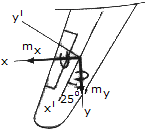The main beam along the wing of an airplane is swept back at an angle of 25°. From load calculations it is determined that the beam is subjected to couple moments aMx = 25,000 lb • ft and My = 17,000 lb • ft. Determine the equivalent couple moments created about the x' and y' axis.

 A. Mx' = 26.0 kip-ft, My' = 29.8 kip-ft B. Mx' = 29.8 kip-ft, My' = -4.84 kip-ft C. Mx' = 26.0 kip-ft, My' = 15.47 kip-ft D. Mx' = 29.8 kip-ft, My' = 4.84 kip-ft

Explanation:

No answer description available for this question. Let us discuss.

2.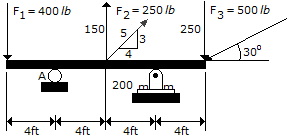Determine the moment of force F1 about point A on the beam.

 A. M1 = 1600 ft-lb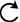B. M1 = 100 ft-lbC. M1 = 100 ft-lb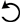D. M1 = 1600 ft-lbExplanation:

No answer description available for this question. Let us discuss.

3.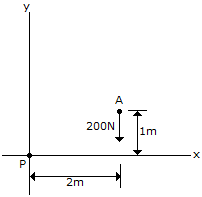Determine the magnitude and direction of the moment of the force of the movement if the force at A about point P.

 A. Mp = 400 NmB. Mp = 400 NmC. Mp = 200 NmD. Mp = 200 NmExplanation:

No answer description available for this question. Let us discuss.

4.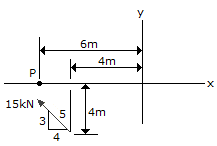Replace the force at A by an equivalent force and couple moment at P.

 A. F = 15 kN @= 36.9°, Mp = 67.1 kN-mB. F = 15 kN @= 36.9°, Mp = 30 kN-mC. F = 15 kN @= 143.1°, Mp = 67.1 kN-mD. F = 15 kN @= 143.1°, Mp = 30 kN-mExplanation:

No answer description available for this question. Let us discuss.

5.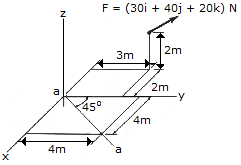Determine the magnitude of the projection of the moment cause by the force about the aa axis.

 A. Maa = 80.0 N-m B. Maa = 56.6 N-m C. Maa = 28.3 N-m D. Maa = 100.0 N-m